# Laplace-Beltrami equation

(Redirected from Laplace–Beltrami equation)

Beltrami equation

A generalization of the Laplace equation for functions in a plane to the case of functionson an arbitrary two-dimensional Riemannian manifoldof class. For a surfacewith local coordinatesand first fundamental form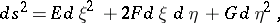the Laplace–Beltrami equation has the form(*)

Forand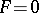, that is, when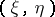are isothermal coordinates on, equation (*) becomes the Laplace equation. The Laplace–Beltrami equation was introduced by E. Beltrami in 1864–1865 (see ).

The left-hand side of equation (*) divided byis called the second Beltrami differential parameter.

Regular solutionsof the Laplace–Beltrami equation are generalizations of harmonic functions and are usually called harmonic functions on the surface(cf. also Harmonic function). These solutions are interpreted physically like the usual harmonic functions, e.g. as the velocity potential of the flow of an incompressible liquid flowing over the surface, or as the potential of an electrostatic field on, etc. Harmonic functions on a surface retain the properties of ordinary harmonic functions. A generalization of the Dirichlet principle is valid for them: Among all functionsof class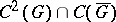in a domainthat take the same values on the boundaryas a harmonic function, the latter gives the minimum of the Dirichlet integral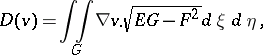where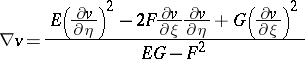is the first Beltrami differential parameter, which is a generalization of the square of the gradientto the case of functions on a surface.

For generalizations of the Laplace–Beltrami equation to Riemannian manifolds of higher dimensions see Laplace operator.